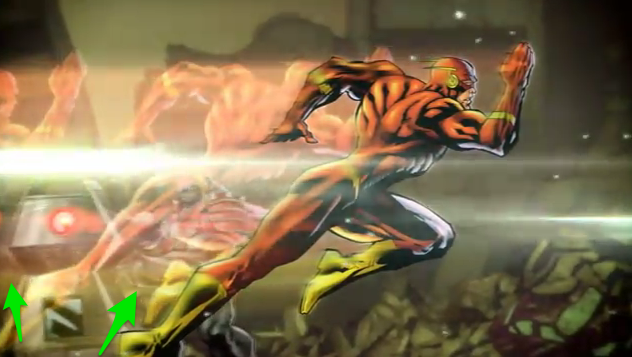# Any idea on this flash/charge effect

Hello there,

I’m trying to make a similar effect like in the visual below. There are 2-3 flash characters appearing and fading out while flash is running. Green arrows on the image is showing what I mean. Is it possible to do similar kind of effect on characters without consuming too much CPU ?Helps are greatly appreciated

Thanks

Here is some code that uses quads in a mesh rather than individual game objects to create the duplicates. I found the setup fussy and required a trail renderer as well to make things look right. It uses two AnimationCurves…one to define how the duplicates space out, and one to define the transparency. Note the code requires a shader that supports vertex colors. Rather than give a detailed explanation of the setup, you will find a Unity Package here:

``````using UnityEngine;
using System.Collections;

public Material material;
public AnimationCurve acTransparency;
public AnimationCurve acSpacing;
public float spacingFactor = 0.5f;
public float speed = 12.0f;

private Mesh mesh;
private float[] lerpingFactor;
private Vector3[] offsets;
private Vector3 prevPos;

private Vector3[] vertices;

private Vector3 v0 = new Vector3(-0.5f, 0.5f, 0);
private Vector3 v1 = new Vector3(-0.5f,-0.5f, 0);
private Vector3 v2 = new Vector3( 0.5f,-0.5f, 0);
private Vector3 v3 = new Vector3( 0.5f, 0.5f, 0);

void Start () {

for (int i = 0; i < quadCount; i++) {
lerpingFactor _= acSpacing.Evaluate ((float)i / (quadCount - 1.0f)) * speed;_
``````
• `````` }*
``````
• `````` MeshFilter mf = GetComponent<MeshFilter>();*
``````
• `````` if (mf == null) {*
``````
• `````` 	mf = gameObject.AddComponent<MeshFilter>();*
``````
• `````` }*
``````
• `````` MeshRenderer mr = gameObject.AddComponent<MeshRenderer>();*
``````
• `````` mr.material = material;*
``````
• `````` mesh = new Mesh();*
``````
• `````` mesh.MarkDynamic();*
``````
• `````` mf.mesh = mesh;*
``````

_ vertices = new Vector3[quadCount * 4];_
_ int triangles = new int[quadCount * 2 * 3];_
_ Color colors = new Color[quadCount * 4];_
_ Vector2 uvs = new Vector2[quadCount * 4];_

• `````` for (int j = 0; j < quadCount; j++) {*
``````

_ vertices[j * 4 ] = v0;_
_ vertices[j * 4 + 1] = v1;_
_ vertices[j * 4 + 2] = v2;_
_ vertices[j * 4 + 3] = v3;_

• `````` }*
``````
• `````` for (int j = 0; j < quadCount; j++) {*
``````

triangles[j * 6 + 0] = j * 4 + 0; // 0_ 3 0 ___ 3
_ triangles[j * 6 + 1] = j * 4 + 3; // | / | /|_
triangles[j * 6 + 2] = j * 4 + 1; // 1|/ 1|/__|2

_ triangles[j * 6 + 3] = j * 4 + 3; // 3_
_ triangles[j * 6 + 4] = j * 4 + 2; // /|_
triangles[j * 6 + 5] = j * 4 + 1; // 1/_|2

• `````` }*
``````
• `````` for (int j = 0; j < vertices.Length / 4; j++) {*
``````

_ uvs[j * 4 + 0] = new Vector2(0,1);_
_ uvs[j * 4 + 1] = new Vector2(0,0);_
_ uvs[j * 4 + 2] = new Vector2(1,0);_
_ uvs[j * 4 + 3] = new Vector2(1,1);_

• `````` }*
``````
• `````` Color c = Color.white;*
``````
• `````` for (int i = 0; i < quadCount; i++) {*
``````
• `````` 	c.a = acTransparency.Evaluate ((float)i/(quadCount - 1.0f));*
``````

_ colors[i * 4 ] = c;_
_ colors[i * 4 + 1] = c;_
_ colors[i * 4 + 2] = c;_
_ colors[i * 4 + 3] = c;_

• `````` }*
``````
• `````` mesh.vertices = vertices;*
``````
• `````` Debug.Log (vertices.Length);*
``````
• `````` mesh.triangles = triangles;*
``````
• `````` mesh.colors = colors;*
``````
• `````` mesh.uv = uvs;*
``````
• `````` mesh.RecalculateNormals();*
``````
• `````` prevPos = transform.position;*
``````
• }*

• void Update () {*

• `````` Vector3 delta = prevPos - transform.position;*
``````
• `````` prevPos = transform.position;*
``````
• `````` for (int i = 0; i < quadCount; i++) {*
``````

_ offsets += delta;_
offsets = Vector3.MoveTowards (offsets_, Vector3.zero, Time.deltaTime * lerpingFactor*);
vertices[i * 4 ] = offsets + v0;
vertices[i * 4 + 1] = offsets + v1;
vertices[i * 4 + 2] = offsets + v2;
vertices[i * 4 + 3] = offsets + v3;
}
mesh.vertices = vertices;
}
}
If your original object is 2D, it would be possible to fold the display of the original plus the the display of the trail into this code, so the result would be one mesh for everything (original object, duplicates and trail), which would draw in a single draw call.
: Welcome laughingloops.com - Hostmonster.com

There are shaders like the Silhouette Outline one on the Unity Wiki that show a copy of the mesh, maybe you can hack one of these so it shows two or three copies of the mesh offset to the back of the object.